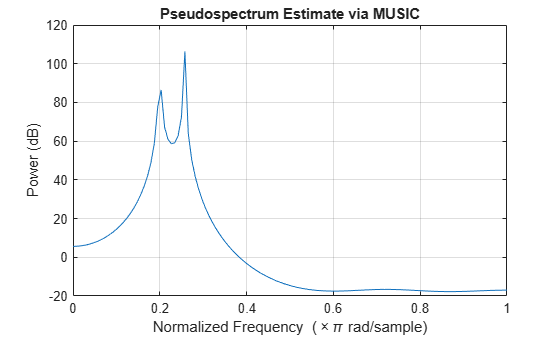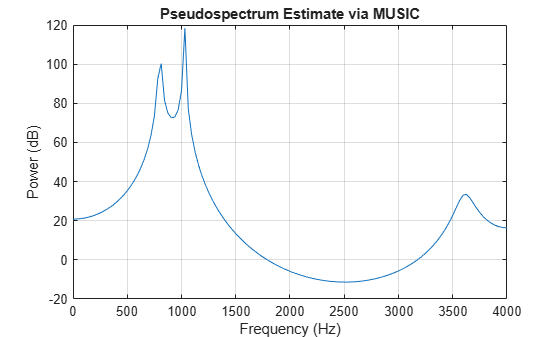Main Content

# pmusic

Pseudospectrum using MUSIC algorithm

## Syntax

``[S,wo] = pmusic(x,p)``
``[S,wo] = pmusic(x,p,wi)``
``[S,wo] = pmusic(___,nfft)``
``[S,wo] = pmusic(___,'corr')``
``[S,fo] = pmusic(x,p,nfft,fs)``
``[S,fo] = pmusic(x,p,fi,fs)``
``[S,fo] = pmusic(x,p,nfft,fs,nwin,noverlap)``
``[___] = pmusic(___,freqrange)``
``[___,v,e] = pmusic(___) ``
``pmusic(___)``

## Description

example

````[S,wo] = pmusic(x,p)` implements the multiple signal classification (MUSIC) algorithm and returns `S`, the pseudospectrum estimate of the input signal `x`, and a vector `wo` of normalized frequencies (in rad/sample) at which the pseudospectrum is evaluated. You can specify the signal subspace dimension using the input argument `p`.```
````[S,wo] = pmusic(x,p,wi)` returns the pseudospectrum computed at the normalized frequencies specified in vector `wi`. The vector `wi` must have two or more elements, because otherwise the function interprets it as `nfft`.```
````[S,wo] = pmusic(___,nfft)` specifies the integer length of the FFT, `nfft`, used to estimate the pseudospectrum. This syntax can include any combination of input arguments from previous syntaxes.```

example

````[S,wo] = pmusic(___,'corr')` forces the input argument `x` to be interpreted as a correlation matrix rather than matrix of signal data. For this syntax, `x` must be a square matrix, and all of its eigenvalues must be nonnegative.```

example

````[S,fo] = pmusic(x,p,nfft,fs)` returns the pseudospectrum computed at the frequencies specified in vector `fo` (in Hz). Supply the sample rate `fs` in Hz.```
````[S,fo] = pmusic(x,p,fi,fs)` returns the pseudospectrum computed at the frequencies specified in the vector `fi`. The vector `fi` must have two or more elements, because otherwise the function interprets it as `nfft`.```

example

````[S,fo] = pmusic(x,p,nfft,fs,nwin,noverlap)` returns the pseudospectrum `S` by segmenting the input data `x` using the window `nwin` and overlap length `noverlap`.```
````[___] = pmusic(___,freqrange)` specifies the range of frequency values to include in `fo` or `wo`. ```
````[___,v,e] = pmusic(___) `returns the matrix `v` of noise eigenvectors along with the associated eigenvalues in the vector `e`.```

example

````pmusic(___)` with no output arguments plots the pseudospectrum in the current figure window.```

## Examples

collapse all

This example analyzes a signal vector, `x`, assuming that two real sinusoidal components are present in the signal subspace. In this case, the dimension of the signal subspace is 4, because each real sinusoid is the sum of two complex exponentials.

```n = 0:199; x = cos(0.257*pi*n) + sin(0.2*pi*n) + 0.01*randn(size(n)); pmusic(x,4) % Set p to 4 because there are two real inputs```This example analyzes the same signal vector, `x`, with an eigenvalue cutoff of 10% above the minimum. Setting `p(1) = Inf` forces the signal/noise subspace decision to be based on the threshold parameter, `p(2)`. Specify the eigenvectors of length 7 using the `nwin` argument, and set the sampling frequency, `fs`, to 8 kHz:

```rng default n = 0:199; x = cos(0.257*pi*n) + sin(0.2*pi*n) + 0.01*randn(size(n)); [P,f] = pmusic(x,[Inf,1.1],[],8000,7); % Window length = 7 plot(f,20*log10(abs(P))) xlabel 'Frequency (Hz)', ylabel 'Power (dB)' title 'Pseudospectrum Estimate via MUSIC', grid on```Supply a positive definite correlation matrix, `R`, for estimating the spectral density. Use the default 256 samples.

```R = toeplitz(cos(0.1*pi*(0:6))) + 0.1*eye(7); pmusic(R,4,'corr')```Enter a signal data matrix, `Xm`, generated from data using `corrmtx`.

```n = 0:699; x = cos(0.257*pi*(n)) + 0.1*randn(size(n)); Xm = corrmtx(x,7,'modified'); pmusic(Xm,2)```Use the same signal, but let `pmusic` form the 100-by-7 data matrix using its windowing input arguments. In addition, specify an FFT of length 512.

```n = 0:699; x = cos(0.257*pi*(n)) + 0.1*randn(size(n)); [PP,ff] = pmusic(x,2,512,[],7,0); pmusic(x,2,512,[],7,0)```## Input Arguments

collapse all

Input signal, specified as a vector or matrix. If `x` is a vector, then it is treated as one observation of the signal. If `x` is a matrix, each row of `x` represents a separate observation of the signal. For example, each row is one output of an array of sensors, as in array processing, such that `x'*x` is an estimate of the correlation matrix.

Note

You can use the output of `corrmtx` to generate `x`.

Complex Number Support: Yes

Subspace dimension, specified as a real positive integer or a two-element vector. If `p` is a real positive integer, then it is treated as the subspace dimension. If `p` is a two-element vector, the second element of `p` represents a threshold that is multiplied by λmin, the smallest estimated eigenvalue of the signal's correlation matrix. Eigenvalues below the threshold λmin*`p(2)` are assigned to the noise subspace. In this case, `p(1)` specifies the maximum dimension of the signal subspace. The extra threshold parameter in the second entry in `p` provides you more flexibility and control in assigning the noise and signal subspaces.

Note

If the inputs to `peig` are real sinusoids, set the value of `p` to double the number of sinusoids. If the inputs are complex sinusoids, set `p` equal to the number of sinusoids.

Complex Number Support: Yes

Input normalized frequencies, specified as a vector.

Data Types: `double`

Number of DFT points, specified as a positive integer. If `nfft` is specified as empty, the default `nfft` is used.

Sample rate, specified as a positive scalar in Hz. in Hz. If you specify `fs` with the empty vector [], the sample rate defaults to `1` Hz.

Input frequencies, specified as a vector. The pseudospectrum is computed at the frequencies specified in the vector.

Length of rectangular window, specified as a nonnegative integer.

Number of overlapped samples, specified as a nonnegative integer smaller than the length of window.

Note

The arguments `nwin` and `noverlap` are ignored when you include `'corr'` in the syntax.

Frequency range of pseudospectrum estimates,specified as one of `'half'`, `whole`, or `'centered'`.

• `'half'` — Returns half the spectrum for a real input signal `x`. If `nfft` is even, then `S` has length `nfft`/2 + 1 and is computed over the interval [0, π]. If `nfft` is odd, the length of `S` is (`nfft` + 1)/2 and the frequency interval is [0,π). When your specify `fs`, the intervals are [0, `fs`/2) and [0, `fs`/2] for even and odd `nfft`, respectively.

• `'whole'` — Returns the whole spectrum for either real or complex input `x`. In this case, `S` has length `nfft` and is computed over the interval [0, 2π). When you specify `fs`, the frequency interval is [0, `fs`).

• `'centered'` — Returns the centered whole spectrum for either real or complex input `x`. In this case, `S` has length `nfft` and is computed over the interval (–π, π] for even `nfft` and (–π, π) for odd `nfft`. When you specify `fs`, the frequency intervals are (–`fs`/2, `fs`/2] and (–`fs`/2, `fs`/2) for even and odd `nfft`, respectively.

Note

You can put the arguments `freqrange` or `'corr'` anywhere in the input argument list after `p`.

## Output Arguments

collapse all

Pseudospectrum estimate, returned as a vector. The pseudospectrum is calculated using estimates of the eigenvectors of a correlation matrix associated with the input data `x`.

Output normalized frequencies, specified as a vector. `S` and `wo` have the same length. In general, the length of the FFT and the values of the input `x` determine the length of the computed `S` and the range of the corresponding normalized frequencies. The table indicates the length of `S` (and `wo`) and the range of the corresponding normalized frequencies for the first syntax.

S Characteristics for an FFT Length of 256 (Default)

Input Data TypeLength of S and w0Range of the Corresponding Normalized Frequencies

Real

129

[0, π]

Complex

256

[0, 2π)

If `nfft` is specified, the following table indicates the length of `S` and `wo` and the frequency range for `wo`.

S and Frequency Vector Characteristics

Input Data Typenfft Even or OddLength of S and wRange of w

Real

Even

`(nfft/2 )+ 1`

[0, π]

Real

Odd

`(nfft + 1)/2`

[0, π)

Complex

Even or odd

`nfft`

[0, 2π)

Output frequency, returned as a vector. The frequency range for `fo` depends on `nfft`, `fs`, and the values of the input `x`. The length of `S` (and `fo`) is the same as in the S and Frequency Vector Characteristics above. The following table indicates the frequency range for `fo` if `nfft` and `fs` are specified.

S and Frequency Vector Characteristics with fs Specified

Input Data Type

nfft Even/Odd

Range of f

Real

Even

[0, `fs`/2]

Real

Odd

[0, `fs`/2)

Complex

Even or odd

[0, `fs`)

Additionally, if `nwin` and `noverlap` are also specified, the input data `x` is segmented and windowed before the matrix used to estimate the correlation matrix eigenvalues is formulated. The segmentation of the data depends on `nwin`, `noverlap`, and the form of `x`. Comments on the resulting windowed segments are described in the following table.

Windowed Data Depending on x and nwin

form of x

Form of nwin

Windowed Data

Data vector

Scalar

Length is `nwin`.

Data vector

Vector of coefficients

Length is `length(nwin)`.

Data matrix

Scalar

Data is not windowed.

Data matrix

Vector of coefficients

`length(nwin)` must be the same as the column length of `x`, and `noverlap` is not used.

See the Eigenvector Length Depending on Input Data and Syntax for related information on this syntax.

Noise eigenvectors, returned as a matrix. The columns of `v` span the noise subspace of dimension `size(v,2)`. The dimension of the signal subspace is `size(v,1)-size(v,2)`.

Estimated eigenvalues of the correlation matrix, returned as a vector.

## Tips

In the process of estimating the pseudospectrum, `pmusic` computes the noise and signal subspaces from the estimated eigenvectors vj and eigenvalues λj of the signal's correlation matrix. The smallest of these eigenvalues is used in conjunction with the threshold parameter `p(2)` to affect the dimension of the noise subspace in some cases.

The length n of the eigenvectors computed by `pmusic` is the sum of the dimensions of the signal and noise subspaces. This eigenvector length depends on your input (signal data or correlation matrix) and the syntax you use.

The following table summarizes the dependency of the eigenvector length on the input argument.

Eigenvector Length Depending on Input Data and Syntax

Form of Input Data x

Comments on the Syntax

Length n of Eigenvectors

Row or column vector

`nwin` is specified as a scalar integer.

`nwin`

Row or column vector

`nwin` is specified as a vector.

`length(nwin)`

Row or column vector

`nwin` is not specified.

2 × `p(1)`

l-by-m matrix

If `nwin` is specified as a scalar, it is not used. If `nwin` is specified as a vector, `length(nwin)` must equal m.

m

m-by-m nonnegative definite matrix

`'corr'` is specified and `nwin` is not used.

m

You should specify `nwin` > `p(1)` or `length(nwin)` > `p(1)` if you want `p(2)` > `1` to have any effect.

## Algorithms

The multiple signal classification (MUSIC) algorithm estimates the pseudospectrum from a signal or a correlation matrix using Schmidt's eigenspace analysis method . The algorithm performs eigenspace analysis of the signal's correlation matrix to estimate the signal's frequency content. This algorithm is particularly suitable for signals that are the sum of sinusoids with additive white Gaussian noise. The eigenvalues and eigenvectors of the signal's correlation matrix are estimated if you do not supply the correlation matrix.

The MUSIC pseudospectrum estimate is given by

`${P}_{\text{MUSIC}}\left(f\right)=\frac{1}{{e}^{H}\left(f\right)\left(\sum _{k=p+1}^{N}{\text{v}}_{k}{\text{v}}_{k}^{H}\right)e\left(f\right)}=\frac{1}{\sum _{k=p+1}^{N}|{\text{v}}_{k}^{H}e\left(f\right){|}^{2}}$`

where N is the dimension of the eigenvectors and vk is the kth eigenvector of the correlation matrix. The integer p is the dimension of the signal subspace, so the eigenvectors vk used in the sum correspond to the smallest eigenvalues and also span the noise subspace. The vector e(f) consists of complex exponentials, so the inner product

`${v}_{k}^{H}e\left(f\right)$`

amounts to a Fourier transform. This is used for computation of the pseudospectrum estimate. The FFT is computed for each vk and then the squared magnitudes are summed.

 Marple, S. Lawrence. Digital Spectral Analysis. Englewood Cliffs, NJ: Prentice-Hall, 1987, pp. 373–378.

 Schmidt, R. O. “Multiple Emitter Location and Signal Parameter Estimation.” IEEE® Transactions on Antennas and Propagation. Vol. AP-34, March, 1986, pp. 276–280.

 Stoica, Petre, and Randolph L. Moses. Spectral Analysis of Signals. Upper Saddle River, NJ: Prentice Hall, 2005.

## Support

#### Deep Learning for Signal Processing with MATLAB

Download white paper